# How to add White Gaussian Noise to Signal using MATLAB?

A type of noise signal which has a flat power spectral density (PSD) across all frequencies is referred to as white gaussian noise or white noise. In this tutorial, I will explain how to add white gaussian noise to signal using MATLAB. But before that let get a brief overview of white gaussian noise.

## What is White Gaussian Noise?

A type of noise signal which has equal energy at all frequencies within a specified frequency range is termed as white gaussian noise. Therefore, white gaussian noise has a flat power spectral density (PSD) across all frequencies.

In the case of white gaussian noise, the term white indicates that the noise signal has the same properties as the white light has in optics, i.e. contains all visible colors in equal proportions. While gaussian noise indicates that the amplitude of the noise signal follows a gaussian distribution. Where, the gaussian distribution has a bell-shaped curve.

White gaussian noise is used in signal processing to analyze and simulate real world random disturbances that can occur in practical systems.

Now, let us discuss how to add white gaussian noise to a signal using MATLAB.

## Add White Gaussian Noise to Signal

In MATLAB, there is a built-in function 'awgn' that is used to add white gaussian noise to a signal. The step-by-step process to add white gaussian noise to a signal is explained here:

Step (1) – Specify a time vector as per the duration of the input signal. This vector will be used to generate the input signal and also as x-axis in plots.

Step (2) – Use the time vector and generate an input signal of desired wave shape and parameters.

Step (3) – Calculate the signal power.

Step (4) – Set a signal-to-noise ratio (SNR).

Step (5) – Add white gaussian noise to the signal using the 'awgn' function.

Step (6) – Plot the resulting signals.

Now, let us understand these steps practically with the help of examples.

As mentioned earlier, we have a built-in MATLAB function 'awgn' to add white gaussian noise to a signal. However, this function can have various syntax formats based on parameters like noise level, signal power, and more.

The commonly used syntax formats of the 'awgn' function are given below:

• Y = awgn(X, snr)

• Y = awgn(X, snr, signalpower)

• Y = awgn(X, snr, signalpower, randobject)

• Y = awgn(X, snr, signalpower, seed)

• Y = awgn(___, powertype)

Let us discuss each of these syntaxes in detail along with example programs.

## Add White Gaussian Noise to Signal based on Specified SNR

To add white gaussian noise to a signal based on a specified SNR (Signal-to-Noise Ratio), the following syntax of the 'awgn' function is used:

Y = awgn(X, snr);


Here, X is the input signal, snr is the signal to noise ratio, and Y is the noisy signal.

### Example

Here, X is the input signal, snr is the signal to noise ratio, and Y is the noisy signal.

% MATLAB program to add white gaussian noise to signal based on SNR
% Create a sample sinusoidal signal
t = 0:0.005:2;	% Time vector
X = sin(2*pi*5*t);	% Input signal

% Set SNR in dB
snr = 15;

% Add white gaussian noise to signal
Y = awgn(X, snr);

% Plot the original signal and the signal with noise
subplot(2, 1, 2);
plot(t, X, t, Y);
legend('Original Signal', 'Noisy Signal');
title('Input Signal with Noise');


### Output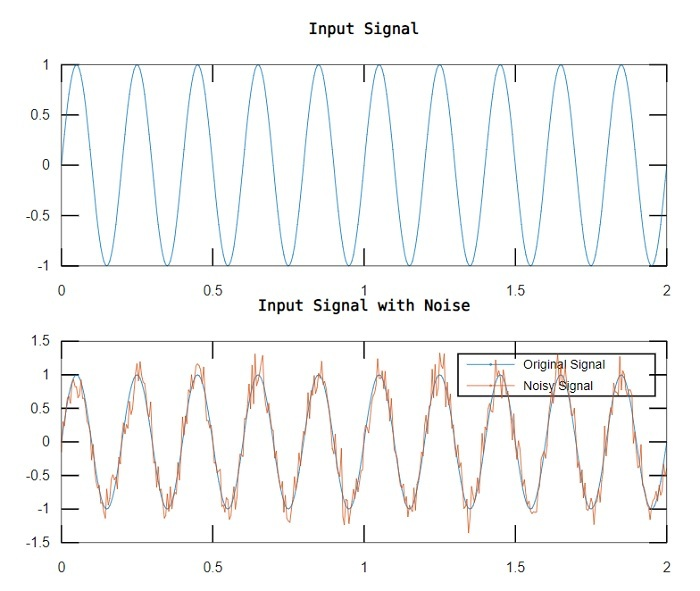## Add White Gaussian Noise to Signal with Specified SNR and Signal Power

The following syntax of the 'awgn' function is used to add white gaussian noise to a signal with specified SNR and signal power:

Y = awgn(X, snr, signalpower);


### Example

Let us see how to implement the code for syntax:

% MATLAB program to add white gaussian noise to signal with specified SNR and signal power
% Generate a sample input signal
t = 0:0.005:2;	% Time vector
X = sin(2*pi*5*t);	% Input signal

% Compute the signal power
signal_power = sum(X.^2) / length(X);

% Set an SNR
snr = 15;

% Add white gaussian noise to signal
Y = awgn(X, snr, signal_power);

% Plot the original signal and the signal with noise
subplot(2, 1, 2);
plot(t, X, t, Y);
legend('Input Signal', 'Noisy Signal');
title('Input Signal with Noise');


### Output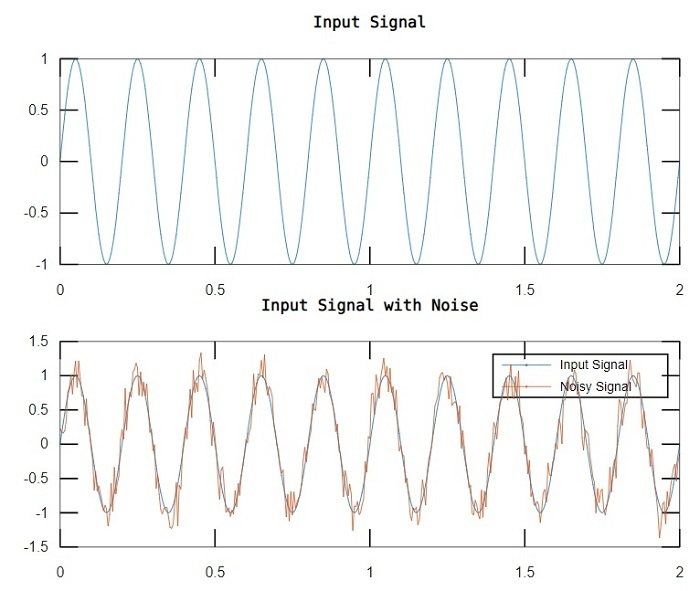## Add White Gaussian Noise to Signal with Specified Random Number Generator Object

The following syntax of the 'awgn' function is used to add white gaussian noise to a signal with a specified random number generator object for generation of normal random noise:

Y = awgn(X, snr, signal_power, rand_object);


### Example

Consider the following MATLAB program to understand code implantation for this syntax:

% MATLAB program to add white gaussian noise to signal with specified random object
% Generate a sample input signal
t = 0:0.005:2;	% Time vector
X = sin(2*pi*5*t);	% Input signal

% Compute the signal power
signal_power = sum(X.^2) / length(X);

% Set an SNR in dB
snr = 15;

% Create a random number generator object
rand_obj = RandStream('mt19937ar', 'Seed', 50);

% Add white gaussian noise to signal using the random object
Y = awgn(X, snr, signal_power, rand_obj);

% Plot the original signal and the signal with noise
subplot(2, 1, 2);
plot(t, X, t, Y);
legend('Input Signal', 'Noisy Signal');
title('Input Signal with Noise');


### Output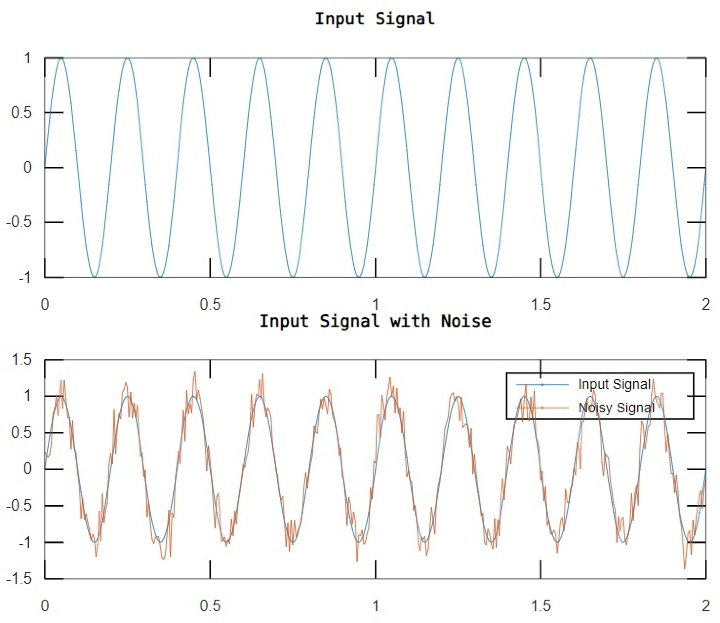## Add White Gaussian Noise to Signal with Specified Random Seed

In MATLAB, we can add white gaussian noise to a signal with a specified random seed for reproducibility by using the following syntax of the 'awgn' function:

Y = awgn(X, snr, signal_power, seed);


### Example

Let's see how to use this function in a MATLAB program.

% MATLAB program to add white gaussian noise to signal with specified random seed
% Generate a sample input signal
t = 0:0.005:2;	% Time vector
X = sin(2*pi*5*t);	% Input signal

% Compute the signal power
signal_power = sum(X.^2) / length(X);

% Set the SNR in dB
snr = 15;

% Set a random seed for reproducibility
seed = 120;

% Add white gaussian noise to signal using the random seed
Y = awgn(X, snr, signal_power, seed);

% Plot the original signal and the signal with noise
subplot(2, 1, 2);
plot(t, X, t, Y);
legend('Input Signal', 'Noisy Signal');
title('Input Signal with Noise');


### Output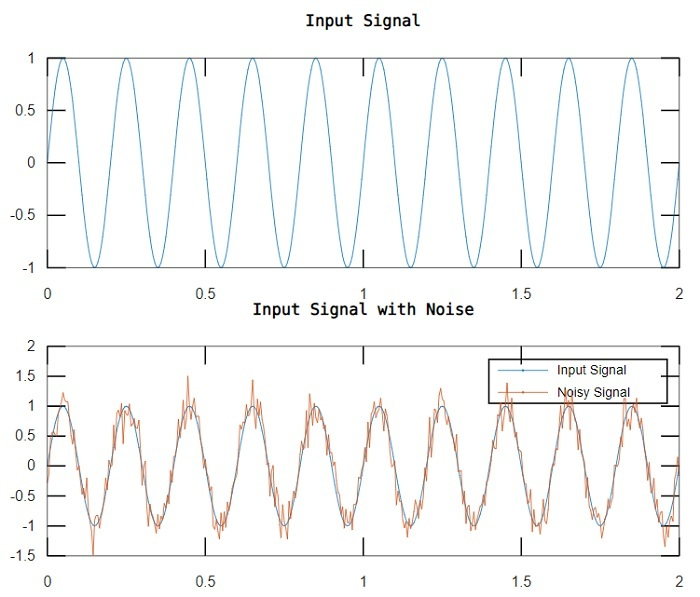## Add White Gaussian Noise to Signal with Specified Power Type

The following syntax of the 'awgn' function is used to add white gaussian noise to a signal with the specified power type:

Y = awgn(X, snr, signal_power, power_type);


Here, the value of the parameter 'power_type' can be 'measured' or 'actual' or 'db'. The default value is 'measured'.

### Example

Let us see an example to understand the code implementation for this syntax.

% MATLAB program to add white gaussian noise to signal with specified power type
% Generate a sample input signal
t = 0:0.005:2;	% Time vector
X = sin(2*pi*5*t);	% Input signal

% Compute the signal power
signal_power = sum(X.^2) / length(X);

% Set the SNR in dB
snr = 15;

% Set the power type
power_type = 'measured';

% Add white gaussian noise to signal using the random seed
Y = awgn(X, snr, signal_power, power_type);

% Plot the original signal and the signal with noise
subplot(2, 1, 2);
plot(t, X, t, Y);
legend('Input Signal', 'Noisy Signal');
title('Input Signal with Noise');


### Output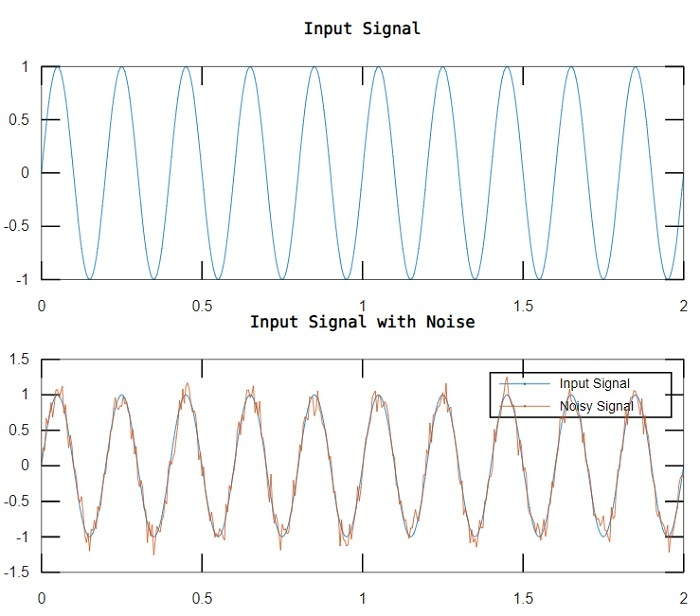## Conclusion

This is all about adding white gaussian noise to a signal using MATLAB. In MATLAB, a built-in function 'awgn' is used to add the white gaussian noise to a signal. In this tutorial, I explained all the possible syntax formats of the 'awgn' function with the help of example programs.

Updated on: 07-Sep-2023

140 Views